SBOS695A August   2014  – December 2014

PRODUCTION DATA.

1. Features
2. Applications
3. Description
4. Revision History
5. Device Comparison Table
6. Pin Configuration and Functions
7. Specifications
8. Parameter Measurement Information
9. Detailed Description
1. 9.1 Overview
2. 9.2 Functional Block Diagram
3. 9.3 Feature Description
4. 9.4 Device Functional Modes
10. 10Application and Implementation
1. 10.1 Application Information
2. 10.2 Typical Application
1. 10.2.1 Design Requirements
2. 10.2.2 Detailed Design Procedure
3. 10.3 Do's and Don'ts
11. 11Power-Supply Recommendations
12. 12Layout
13. 13Device and Documentation Support
1. 13.1 Device Support
2. 13.2 Documentation Support
4. 13.4 Electrostatic Discharge Caution
5. 13.5 Glossary
14. 14Mechanical, Packaging, and Orderable Information

• RMS|14

## 7 Specifications

### 7.1 Absolute Maximum Ratings

over operating free-air temperature range (unless otherwise noted)(1)
MIN MAX UNIT
Voltage Power supply 5.5 V
Input voltage range VS– – 0.7 VS+ + 0.7 V
Current Input current, IN+, IN– 10 mA
Output current (sourcing or sinking) OUT+, OUT– 100 mA
Continuous power dissipation See Thermal Information
Temperature Maximum junction temperature, TJ 150 °C
Maximum junction temperature, continuous operation, long-term reliability 125 °C
Operating free-air, TA –40 85 °C
Storage, Tstg –40 150 °C
(1) Stresses beyond those listed under Absolute Maximum Ratings may cause permanent damage to the device. These are stress ratings only, which do not imply functional operation of the device at these or any other conditions beyond those indicated under Recommended Operating Conditions. Exposure to absolute-maximum-rated conditions for extended periods may affect device reliability.

### 7.2 ESD Ratings

VALUE UNIT
V(ESD) Electrostatic discharge Human body model (HBM), per ANSI/ESDA/JEDEC JS-001(1) ±2500 V
Charged device model (CDM), per JEDEC specification JESD22-C101(2) ±1000
(1) JEDEC document JEP155 states that 500-V HBM allows safe manufacturing with a standard ESD control process.
(2) JEDEC document JEP157 states that 250-V CDM allows safe manufacturing with a standard ESD control process.

### 7.3 Recommended Operating Conditions

over operating free-air temperature range (unless otherwise noted)
MIN NOM MAX UNIT
Supply voltage (VS = VS+ – VS–) 3.15 5 5.25 V
Operating junction temperature, TJ –40 125 °C
Ambient operating air temperature, TA –40 25 85 °C

### 7.4 Thermal Information

THERMAL METRIC(1) LMH3401 UNIT
RMS (UQFN)
14 PINS
RθJA Junction-to-ambient thermal resistance 101 °C/W
RθJC(top) Junction-to-case (top) thermal resistance 51
RθJB Junction-to-board thermal resistance 61
ψJT Junction-to-top characterization parameter 4.2
ψJB Junction-to-board characterization parameter 61
RθJC(bot) Junction-to-case (bottom) thermal resistance N/A

### 7.5 Electrical Characteristics: VS = 5 V

Test conditions are at TA = 25°C, VS+ = 2.5 V, VS– = –2.5 V, VCM = 0 V, RL = 200-Ω differential, G = 16 dB, single-ended input and differential output, and input and output referenced to midsupply, unless otherwise noted. Measured using an evaluation module (EVM) as discussed in the Parameter Measurement Information section.
PARAMETER TEST CONDITIONS MIN TYP MAX UNIT TEST
LEVEL(1)
AC PERFORMANCE
Small-signal bandwidth VO = 200 mVPP(3) 7 GHz C
Large-signal bandwidth VO = 2 VPP 4 GHz C
Bandwidth for 0.1-dB flatness VO = 2 VPP 700 MHz C
Slew rate VO = 2-V step 18000 V/µs C
Rise time VO = 1-V step 80 ps C
Fall time VO = 1-V step 80 ps C
Settling time to 1% VO = 2-V step 1 ns C
Input return loss, s11 See S-Parameters section, f < 1 GHz –20 dB C
Output return loss, s22 See S-Parameters section, f < 1 GHz –20 dB C
Reverse isolation, s12 See S-Parameters section, f < 1 GHz –65 dB C
Second-order harmonic distortion f = 10 MHz, VO = 2 VPP –96 dBc C
f = 500 MHz, VO = 2 VPP –79 dBc C
f = 1 GHz, VO = 2 VPP –64 dBc C
f = 2 GHz, VO = 2 VPP –55 dBc C
Third-order harmonic distortion f = 10 MHz, VO = 2 VPP –102 dBc C
f = 500 MHz, VO = 2 VPP –77 dBc C
f = 1 GHz, VO = 2 VPP –72 dBc C
f = 2 GHz, VO = 2 VPP –40 dBc C
Second-order intermodulation distortion f = 10 MHz, VO = 1 VPP per tone –90 dBc C
f = 500 MHz, VO = 1 VPP per tone –77 dBc C
f = 1 GHz, VO = 1 VPP per tone –71 dBc C
f = 2 GHz, VO = 1 VPP per tone –56 dBc C
Third-order intermodulation distortion f = 10 MHz, VO = 1 VPP per tone –101 dBc C
f = 500 MHz, VO = 1 VPP per tone –86 dBc C
f = 1 GHz, VO = 1 VPP per tone –73 dBc C
f = 2 GHz, VO = 1 VPP per tone –52 dBc C
1-dB compression point f = 200 MHz, power measured at amplifier 13 dBm C
Output third-order intercept point At device outputs, f = 200 MHz 45 dBm C
At device outputs, f = 1000 MHz 33 dBm C
Input-referred voltage noise f > 1 MHz 1.4 nV/√Hz C
Noise figure 50-Ω, single-ended source f = 200 MHz 9 dB C
f = 1 GHz 9.4 dB C
Overdrive recovery Overdrive = ±0.5 V 300 ps C
Output balance error f = 1000 MHz 45 dBc C
Output impedance At dc 16 20 24 Ω A
DC PERFORMANCE
Gain 50-Ω single-ended source, with external 50-Ω termination 15.4 16 16.6 dB A
100-Ω differential source, external termination 12 dB C
Differential output offset TA = 25°C ±2 ±20 mV A
TA = –40°C to 85°C ±4 mV C
Differential output offset temperature drift 4 µV/°C C
Common-mode rejection ratio TA = 25°C 72 dBc C
INPUT
Differential input resistance 22 25 29 Ω A
Single ended input resistance With external 50-Ω resistor on INN to ground 45 50 55 Ω A
Input common-mode range low Inputs shorted together, VCM = 2.5 V VS– – 0.7 VS– + 0.2 V A
Input common-mode range high Inputs shorted together, VCM = 2.5 V VS+ – 1.3 VS+ – 1.2 V A
OUTPUT
Output voltage range high Measured single-ended TA = 25°C VS+ – 1.3 VS+ – 1.1 V A
TA = –40°C to 85°C VS+ – 1.2 V C
Output voltage range low Measured single-ended TA = 25°C VS– + 1.3 VS– + 1.1 V A
TA = –40°C to 85°C VS– + 1.2 V C
Differential output voltage 5.6 VPP C
Differential output current drive VO = 0 V 40 50 mA A
OUTPUT COMMON-MODE VOLTAGE CONTROL
VCM small-signal bandwidth VOUT_CM = 200 mVPP 3.3 GHz C
VCM slew rate VOUT_CM = 500 mVPP 2900 V/µs C
VCM voltage range low Differential gain shift < 1 dB VS– + 1.6 VS– + 2.0 V A
VCM voltage range high Differential gain shift < 1 dB VS+ – 2.0 VS+ – 1.6 V A
VCM gain VCM = 0 V 0.98 1.0 1.01 V/V A
VOUT_CM output common-mode offset
from VCM input voltage (2)
VCM = 0 V –27 mV C
VCM temperature drift –13.6 µV/°C C
POWER SUPPLY
Quiescent current TA = 25°C 50 55 62 mA A
Power-supply rejection ratio VS+ 60 84 dB A
VS– 50 75 dB A
POWER DOWN
Enable or disable voltage threshold Device powers on below 0.8 V,
device powers down above 1.2 V
0.9 1.1 1.2 V A
Power-down quiescent current 1 3 6 mA A
PD bias current PD = 2.5 V 10 ±100 µA C
Turn-on time delay Time to VO = 90% of final value 10 ns C
Turn-off time delay Time to VO = 10% of original value 10 ns C
(1) Test levels: (A) 100% tested at 25°C. Overtemperature limits by characterization and simulation. (B) Limits set by characterization and simulation. (C) Typical value only for information.
(2) VOUT_CM = (OUT+ + OUT–) / 2 and is set by the CM pin VOUT_CM ≈ VCM.
(3) All output voltages are specified as differential voltages unless otherwise noted. Output differential voltage is defined as VO = (VO+ – VO–).

### 7.6 Electrical Characteristics: VS = 3.3 V

Test conditions are at TA = 25°C, VS+ = 1.65 V, VS– = –1.65 V, VCM = 0 V, RL = 200-Ω differential, G = 16 dB, single-ended input and differential output, and input and output referenced to midsupply, unless otherwise noted. Measured using an EVM as discussed in the Parameter Measurement Information section.
PARAMETER TEST CONDITIONS MIN TYP MAX UNIT TEST
LEVEL(1)
AC PERFORMANCE
Small-signal bandwidth VO = 200 mVPP 6.5 GHz C
Large-signal bandwidth VO = 1 VPP 4 GHz C
Bandwidth for 0.1-dB flatness VO = 1 VPP 700 MHz C
Slew rate VO = 1-V step 17600 V/µs C
Rise time VO = 1-V step 90 ps C
Fall time VO = 1-V step 90 ps C
Input return loss, s11 See S-Parameters section, f < 1 GHz –20 dB C
Output return loss, s22 See S-Parameters section, f < 1 GHz –20 dB C
Reverse isolation, s12 See S-Parameters section, f < 1 GHz –65 dB C
Second-order harmonic distortion f = 10 MHz, VO = 1 VPP –97 dBc C
f = 500 MHz, VO = 1 VPP –74 dBc C
f = 1 GHz, VO = 1 VPP –59 dBc C
f = 2 GHz, VO = 1 VPP –48 dBc C
Third-order harmonic distortion f = 10 MHz, VO = 1 VPP –100 dBc C
f = 500 MHz, VO = 1 VPP –66 dBc C
f = 1 GHz, VO = 1 VPP –56 dBc C
f = 2 GHz, VO = 1 VPP –49 dBc C
Second-order intermodulation distortion f = 10 MHz, VO = 0.5 VPP per tone –95 dBc C
f = 500 MHz, VO = 0.5 VPP per tone –81 dBc C
f = 1 GHz, VO = 0.5 VPP per tone –72 dBc C
f = 2 GHz, VO = 0.5 VPP per tone –60 dBc C
Third-order intermodulation distortion f = 10 MHz, VO = 0.5 VPP per tone –100 dBc C
f = 500 MHz, VO = 0.5 VPP per tone –86 dBc C
f = 1 GHz, VO = 0.5 VPP per tone –78 dBc C
f = 2 GHz, VO = 0.5 VPP per tone –56 dBc C
Output third-order intercept point At device outputs, f = 10 MHz 39.5 dBm C
At device outputs, f = 1000 MHz 31 dBm C
Input-referred voltage noise f > 1 MHz 1.4 nV/√Hz C
Noise figure 50-Ω, single-ended source f = 200 MHz 9 dB C
f = 1 GHz 9.4 dB C
Overdrive recovery Overdrive = ±0.5 V 400 ps C
Output impedance f = 100 MHz 16 20 24 Ω A
DC PERFORMANCE
Gain 50-Ω, single-ended source with external 50-Ω termination 15.4 16 16.6 dB A
100-Ω differential source, external termination 12 dB C
Differential output offset voltage TA = 25°C ±2 ±20 mV A
TA = –40°C to 85°C ±4 mV C
Differential output voltage drift 3.6 µV/°C C
Common-mode rejection ratio –72 dB A
INPUT
Differential input resistance 22 25 29 Ω A
Single-ended input resistance With external 50-Ω resistor on INN to ground 45 50 55 Ω A
Input common-mode range low Inputs shorted together VS– – 0.3 VS– + 0.2 V A
Input common-mode range high Inputs shorted together VS+ – 1.5 VS+ –1.6 V A
OUTPUT
Output voltage range high Measured single-ended TA = 25°C VS+ – 1.2 VS+ – 0.95 V A
TA = –40°C to 85°C VS+ – 1.05 V C
Output voltage range low Measured single-ended TA = 25°C VS– + 1.2 VS– + 0.95 V A
TA = –40°C to 85°C VS– + 1.05 V C
Differential output voltage 2.8 VPP C
Differential output current drive VO = 0 V 30 40 mA A
OUTPUT COMMON-MODE VOLTAGE CONTROL
VCM small-signal bandwidth VOUT_CM = 200 mVPP 3 GHz C
VCM slew rate VOUT_CM = 500 mVPP 2600 V/µs C
VCM voltage range low Differential gain shift < 1 dB VS– + 1.35 VS– + 1.55 V A
VCM voltage range high Differential gain shift < 1 dB VS+ – 1.55 VS+ – 1.35 V A
VCM gain VCM = 0 V 0.98 1.0 1.01 V/V A
Output common-mode offset
from VCM input
VCM = 0 V –7 mV C
Common-mode voltage drift –34.6 µV/°C C
POWER SUPPLY
Quiescent current TA = 25°C 49 54 60 mA A
Power-supply rejection ratio VS+ 60 84 dB A
VS– 50 75 dB A
POWER-DOWN
Enable or disable voltage threshold Device powers on below 0.8 V,
device powers down above 1.2 V
1.0 1.1 1.2 V A
Power-down quiescent current 1 1.6 5 mA A
PD bias current PD = 2.5 V 10 ±100 µA C
Turn-on time delay Time to VO = 90% of final value 10 ns C
Turn-off time delay Time to VO = 10% of original value 10 ns C

### 7.7 Typical Characteristics

At TA = 25°C, split supplies, VCM = 0 V, RL = 200-Ω differential (ROUT = 40 Ω each), G = 16 dB, single-ended input and differential output, and input and output pins referenced to midsupply, unless otherwise noted. Measured using an EVM as discussed in the section (see Figure 49 to Figure 53).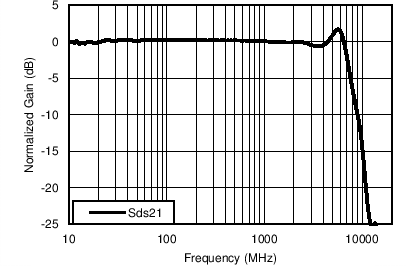VS = ±2.5 V, VOUT_AMP = 0.4 VPP
Figure 1. Frequency Response Single-Ended InputNo input matching resistors, VOUT_AMP = 0.2 VPP, net gain = 14 dB, VS = ±2.5 V
Figure 3. Frequency Response Differential InputVS = ±2.5 V, VOUT_AMP = 4 VPP
Figure 5. Large-Signal Frequency Response
(Single-Ended Input)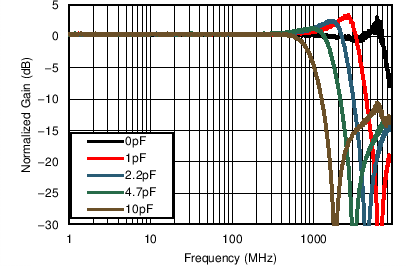VS = ±2.5 V, VOUT_AMP = 1 VPP, capacitance at DUT output pins
Figure 7. Frequency Response with Capacitive Load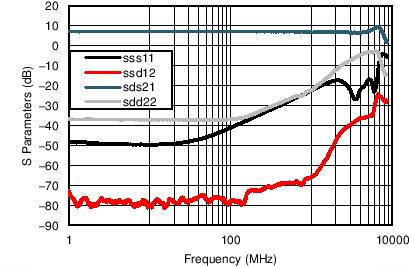VS = ±2.5 V, VOUT_AMP = 200 mVPP
Figure 9. S-Parameters (±2.5-V Supply)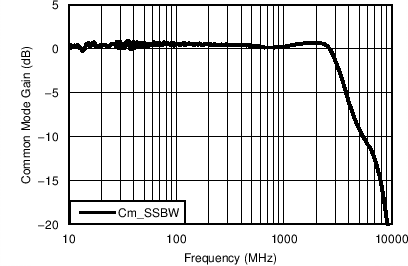VS = ±2.5 V, VOUT_AMP = 100 mVPP
Figure 11. Common-Mode Frequency Response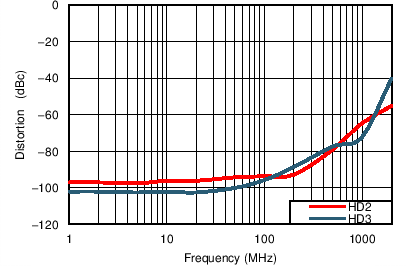VS = ±2.5 V, VOUT_AMP = 2 VPP
Figure 13. HD2 and HD3 (±2.5-V Supply)VS = ±2.5 V, VOUT_AMP = 2 VPP, f = 1 GHz
Figure 15. HD2 and HD3 vs Temperature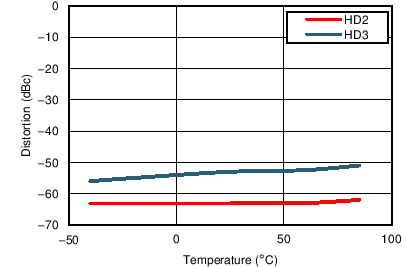VS = ±1.65 V, VOUT_AMP = 1 VPP, f = 1 GHz
Figure 17. HD2 and HD3 vs TemperatureVS = ±1.65 V, f = 200 MHz, VOUT_AMP = 1 VPP
Figure 19. HD2 and HD3 vs Output Common-Mode Voltage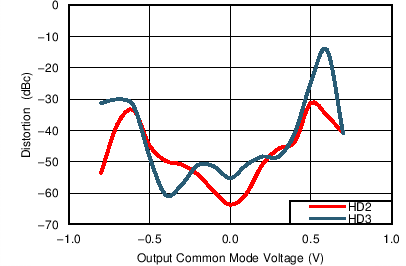VS = ±1.65 V, f = 1000 MHz, VOUT_AMP = 1 VPP
Figure 21. HD2 and HD3 vs Output Common-Mode Voltage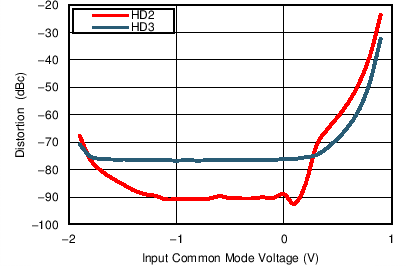VS = ±1.65 V, f = 200 MHz, VOUT_AMP = 1 VPP
Figure 23. HD2 and HD3 vs Input Common-Mode Voltage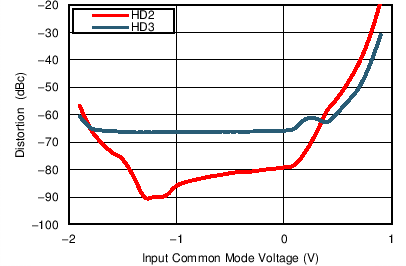VS = ±1.65 V, f = 500 MHz, VOUT_AMP = 1 VPP
Figure 25. HD2 and HD3 vs Input Common-Mode VoltageVS = ±1.65 V, f = 1 GHz, VOUT_AMP = 1 VPP
Figure 27. HD2 and HD3 vs Input Common-Mode Voltage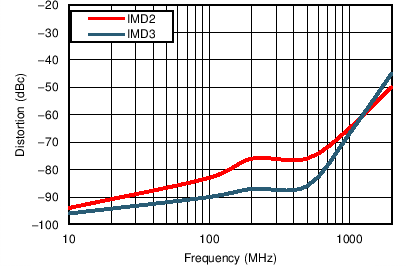VS = ±2.5 V, VOUT_AMP = 1 VPP per tone, RLOAD = 100 Ω
Figure 29. Intermodulation Distortion vs FrequencyVS = ±2.5 V, power measured at amplifier
Figure 31. Intermodulation Distortion (f = 200 MHz)VS = ±2.5 V, power measured at amplifier
Figure 33. Intermodulation Distortion (f = 1000 MHz)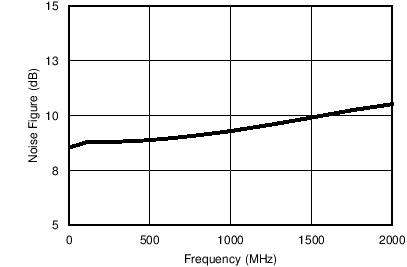VS = ±2.5 V
Figure 35. Noise Figure vs FrequencyVS = ±2.5 V, VOUT_AMP, VCM = (VO+ + VO–) / 2
Figure 37. Pulse Response Common-Mode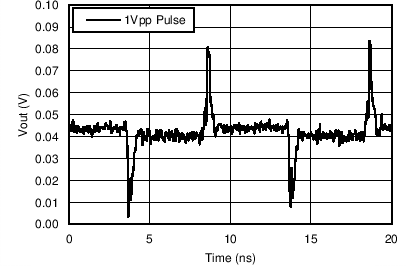VS = ±1.65 V, VOUT_AMP
Figure 39. Pulse Response Common-Mode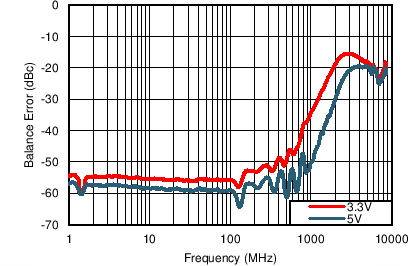Single-ended input, differential output
Figure 41. Balance Error (Scd21)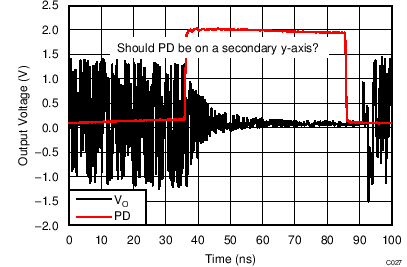VS = ±2.5 V
Figure 43. Power-Down TimingVS = ±2.5 V
Figure 45. Gain Drift vs Temperature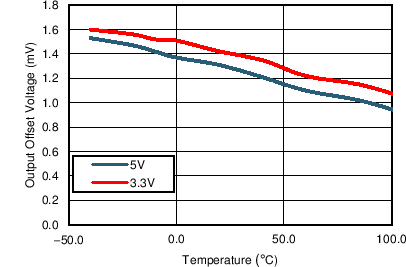At dc
Figure 47. Differential Offset Voltage vs TemperatureExternal 37.5-Ω input matching resistors, VOUT_AMP = 0.4 VPP, gain = 12 dB, see Figure 57, VS = ±1.65 V and ±2.5 V
Figure 2. Frequency Response Differential InputSingle-ended input, VOUT_AMP = 1 VPP
Figure 4. 1-VPP Frequency Response vs Supply Voltage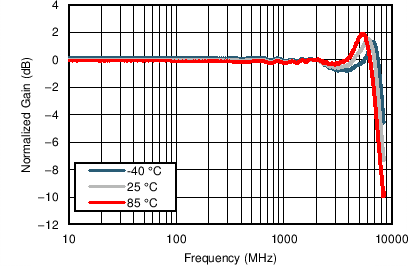VS = ±2.5 V, VOUT_AMP = 1 VPP
Figure 6. Frequency Response vs Temperature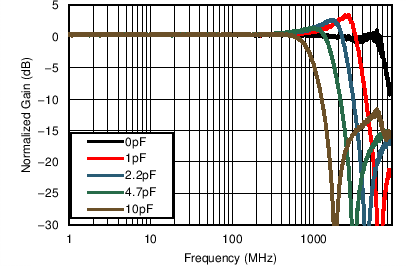VS = ±1.65 V, VOUT_AMP = 1 VPP, capacitance at DUT output pins
Figure 8. Frequency Response with Capacitive Load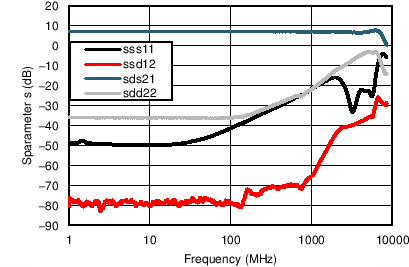VS = ±1.65 V, VOUT_AMP = 200 mVPP
Figure 10. S-Parameters (3.3-V Supply)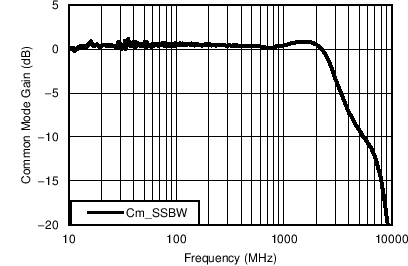VS = ±1.65 V, VOUT_AMP = 100 mVPP
Figure 12. Common-Mode Frequency Response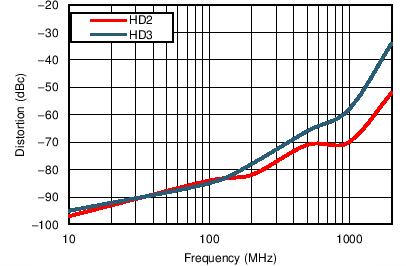VS = ±2.5 V, VOUT_AMP = 2 VPP, RLOAD = 100 Ω
Figure 14. HD2 and HD3 (±2.5-V Supply, 100-Ω Load)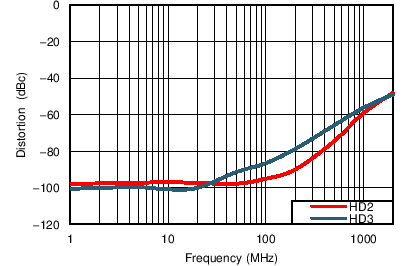VS = ±1.65 V, VOUT_AMP = 1 VPP
Figure 16. HD2 and HD3 (3.3-V VS)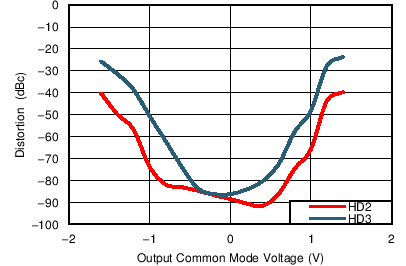VS = ±2.5 V, f = 200 MHz, VOUT_AMP = 2 VPP
Figure 18. HD2 and HD3 vs Output Common-Mode Voltage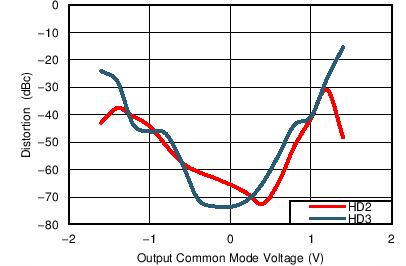VS = ±2.5 V, f = 1000 MHz, VOUT_AMP = 2 VPP
Figure 20. HD2 and HD3 vs Output Common-Mode Voltage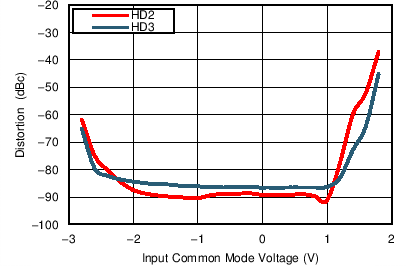VS = ±2.5 V, f = 200 MHz, VOUT_AMP = 2 VPP
Figure 22. HD2 and HD3 vs Input Common-Mode VoltageVS = ±2.5 V, f = 500 MHz, VOUT_AMP = 2 VPP
Figure 24. HD2 and HD3 vs Input Common-Mode Voltage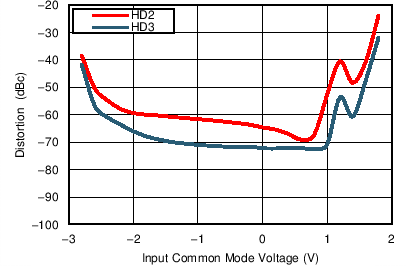VS = ±2.5 V, f = 1 GHz, VOUT_AMP = 2 VPP
Figure 26. HD2 and HD3 vs Input Common-Mode Voltage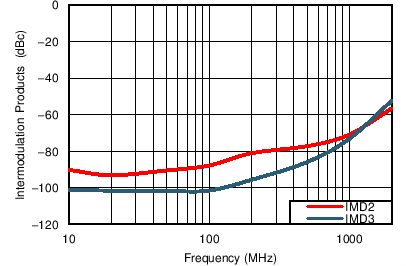VS = ±2.5 V, VOUT_AMP = 1 VPP per tone,
Figure 28. Intermodulation Distortion vs FrequencyVS = ±1.65 V, VOUT_AMP = 0.5 VPP per tone
Figure 30. Intermodulation Distortion vs Frequency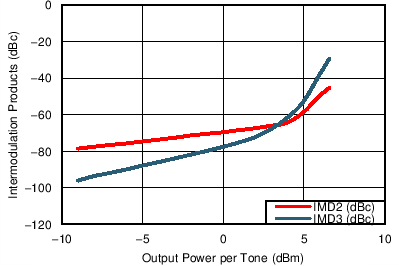VS = ±2.5 V, power measured at amplifier
Figure 32. Intermodulation Distortion (f = 500 MHz)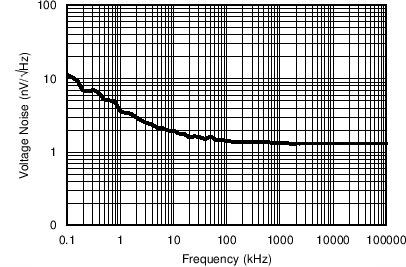VS = ±2.5 V
Figure 34. Input-Referred Voltage NoiseVS = ±2.5 V, VOUT_AMP
Figure 36. Pulse Response for Various VO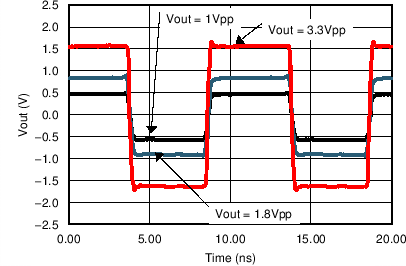VS = ±1.65 V, VOUT_AMP
Figure 38. Pulse Response for Various VODifferential input
Figure 40. CMRR (Sdc21)Common-mode input, common-mode output, RS = 25 Ω, RL = 50 Ω
Figure 42. Common-Mode Frequency Response (Scc21)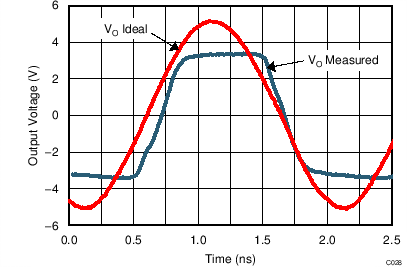VS = ±2.5 V
Figure 44. Overdrive Recovery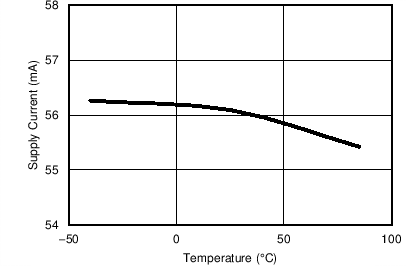VS = ±2.5 V
Figure 46. Supply Current vs Temperature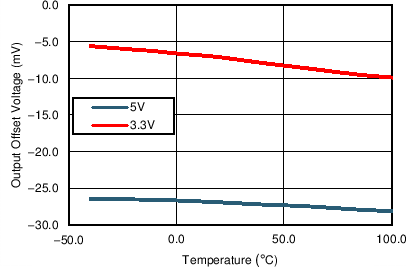At dc
Figure 48. Common-Mode Offset Voltage vs Temperature